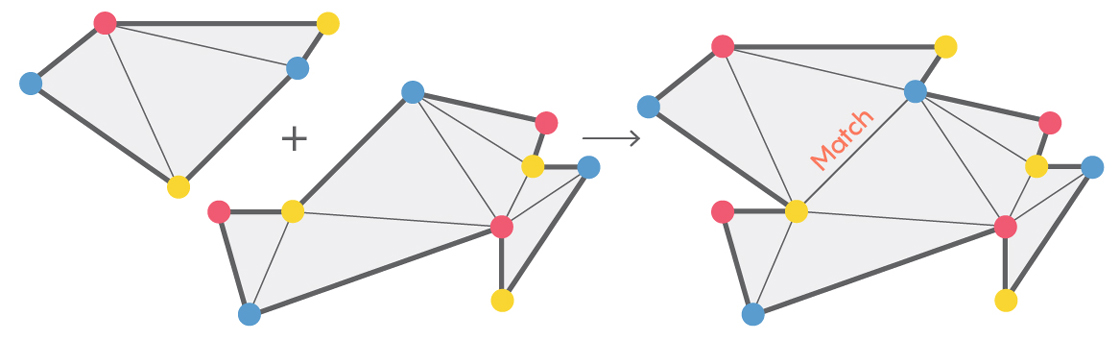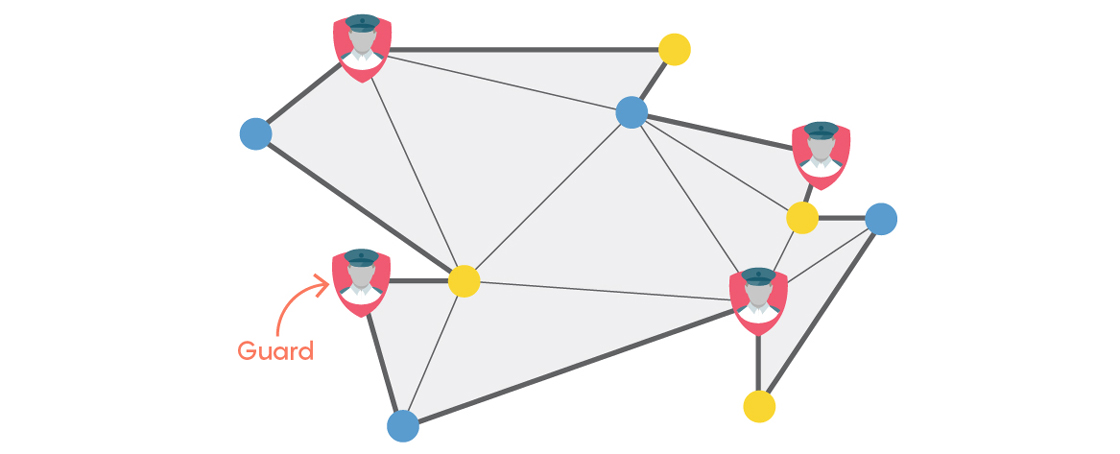March 2018

## The Infinite Primes and Museum Guard Proofs, Explained

### A simple, step-by-step breakdown of two “perfect” math proofs.

#### By Erica Klarreich

In January, I spoke with Günter Ziegler, one of the authors of Proofs From THE BOOK, a compilation of some of the most beautiful and elegant proofs in mathematics. The collection was inspired by the legendary mathematician Paul Erdős, who envisioned an infinite book in which God had written the perfect proof for each theorem. Today I want to share a couple of my favorite proofs from THE BOOK. The first is an old chestnut that all math majors (including this one) bump into sometime during their education; the second came as a delightful surprise.

The volume opens with perhaps the most famous proof in mathematics:

Theorem: There are infinitely many prime numbers.

The proof we’ll give dates back to Euclid, and uses what is, therefore, one of the oldest tricks in the book (and THE BOOK): the notion of a “proof by contradiction.” In such a proof, we assume the opposite of what we really want to prove, and then reason from there until we reach a statement that is clearly impossible.

So let’s assume that there are only finitely many prime numbers. If that’s the case, we can list them all—we’ll name them p1, p2, p3 and so on, all the way through some last prime, pn. Now let’s make a new number, N, by multiplying all these primes together and adding 1:

N = p1p2p3 ... pn + 1.

N cannot be prime, because it’s bigger than any of the numbers on our complete list of primes, and we assumed our list was complete. So must have some factor other than itself and 1, and if we keep dividing that factor into smaller and smaller factors, we’ll eventually hit a prime number p that divides evenly into N.

But which prime number is p? It must be on our list of primes, because our list is complete. But it also can’t be on our list of primes, because none of those primes divide evenly into N: They all leave a remainder of 1. This is a contradiction, so our original assumption—that there are only finitely many primes—must have been wrong. □

(Mathematicians like to put a little box at the end of a proof, to make it easy to spot where the argument ends.)

When I first started studying proofs, I found it somehow unsatisfying that a proof by contradiction doesn’t end by sort of unrolling the contradiction backward, along the lines of “This is a contradiction, so the statement before it must be false, which means the statement before that must be false …” and so on, until we’d worked our way back to the original assumption. But the power of abstraction is precisely that we don’t have to do this each time we prove something by contradiction—we simply understand the underlying principle once, and then we can let it do the heavy lifting in proof after proof.

In our next proof we’ll do something similar, but this time we’re going to abstract the intuition behind the domino effect into a powerful mathematical principle known as induction. This says that to prove a statement about all positive whole numbers, you have to do only two things:

1. Prove that the statement is true for the number 1. (This is called the base case, in which we knock over the first domino.)

2. Prove that whenever the statement is true for a number n, it is also true for n + 1. (This is called the induction step, in which we show that each domino knocks over the next domino.)

Actually, we’re going to use a variation, “strong induction,” in which the induction step requires showing that if the statement we’re trying to prove is true for all numbers from 1 to n, then it’s also true for n + 1. It’s a similar sort of logic to regular induction, but it comes in handy in slightly different settings, including the following:

Theorem: In a museum shaped like a polygon with k walls, there’s always some way to station k/3 or fewer guards so that every spot in the museum is visible to some guard. (So, for example, if our museum has 18 walls, then we need at most 6 guards. If the number of walls isn’t divisible by 3 then we get to round down, so a museum with 19 walls will also need at most 6 guards.)

In the proof that’s contained in THE BOOK, which comes from Steve Fisk, we start by drawing in a bunch of noncrossing diagonals until our museum is divided into triangles:Now we’re going to use strong induction to prove that it’s always possible to color the corners of the room red, yellow and blue so that every color appears exactly once in each triangle. Our induction will be with respect to the number of triangles.

So first we must prove the base case: that we can do such a coloring if our polygon is made of a single triangle. But that’s easy—we can just color the three corners red, yellow. and blue, and we’re done.Now for the induction step: We need to prove that if such a coloring is always possible for any polygon made of one triangle, or two triangles, or three triangles …, all the way to n triangles, then such a coloring is also possible for any polygon made of n + 1 triangles.

So let’s consider a polygon made of n + 1 triangles. We can split it into two smaller polygons by cutting along one of the diagonals:Our induction assumption tells us that each of these smaller polygons can be colored in the desired way. Now to get a coloring of the original “+ 1” polygon, all we have to do is glue the two smaller polygons back together. (If their colorings don’t match along the diagonal where we’re gluing, we can just switch around the reds, yellows and blues in one of the two polygons so it matches the other).That completes our induction argument, so now we know that there’s some way to color the corners of our museum red, yellow, and blue so that all three colors show up in every triangle.

At least one of these colors—let’s say, red—appears on at most k/3 corners, since otherwise the red, yellow, and blue corners would add up to more than k (the total number of corners in the room). Now, station a guard at each red corner. Every point in the museum is in some triangle, so it’s in the sightline of the guard at that triangle’s red corner. □Lead image credit: Aubrey Wade for Quanta Magazine

Reprinted with permission from Quanta Magazine's Abstractions blog.